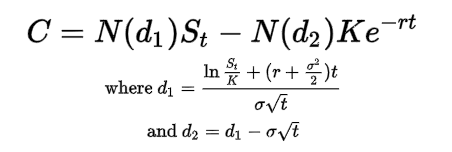# Calculation of Intrinsic Value before expiry?

Hello all,

The formula to calculate the Intrinsic Value of a CE option = Spot - Strike price and that of PE = Strike - Spot.
But this is applicable only on the day of expiry. I want to know how the Intrinsic Value is calculated before expiry. Varsity says the formula is different for Intrinsic Value before expiry. This caused curiosity.
I know the formula for P&L. Just interested for Intrinsic Value (IV).

Thanks

The intrinsic value ( not IV, this is generally used to indicate intrinsic volatility) is spot -price at all times like you indicated. The option price/premium however is a combination of time, volatility as well as intrinsic value amongst other things. This includes the interest rate and rate of dividend that the security may issue. The most popular is the black - scholes model, however there are other models such as the binomial and trinomial models.

The formula for black scholes is :1 Like

Thanks @wabbits bro.